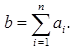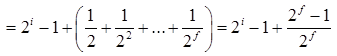# GATE Solved Paper 2017-19 - GATE 2017 Shift 1

>>>>>>>>GATE 2017 Shift 1

• A

a unique solution at x = jn where jn denotes a n-dimensional vector of all 1• B

no solution• C

infinitely many solutions• D

finitely many solutions• Option : C
• Explanation :
Since the scalars are not all zero
∴ The column vectors for i =1,2...,n are linearly dependent
⇒ |A| = 0 and⇒ Ax = b has infinitely many solutions.

• Option : B
• Explanation :
In the given questions
If lists are not NULL : Invocation of join will append list m to the end of list n if the lists are not NULL.
Example:Before join operation :
m =1->2->3->4->5->null
n =6->7->8->9->nullAfter join operation :
6->7->8->9->1->2->3->4->5->null
& If lists are NULL : If the list n is empty and itself NULL, then joining and referencing would obviously create NULL pointer issue.

• A

2-f to 2i• B

2-f to (2i - 2-f)• C

0 to 2i• D

0 to (2i - 2-f)• Option : D
• Explanation :
I=n-f.f
Max value 111.....1 i times .111........1 (f times)= 2-i - 2f
∴ 0 to (2i - 2-f)

• A

p1 = a - b
q 1 = p1 * c
p1 = u * v
q1 = p1 + q1• B

p3 = a - b
q4 = p3 * c
p4 = u * v
q5 = p4 + q4• C

p 1 = a - b
q1 = p2 * c
p3 = u * v
q2 = p4 + q3• D

p1 = a - b
q1 = p * c
p2 = u * v
q2 = p + q• Option : B
• Explanation :
According to Static Single Assignment
--> A variable cannot be used more than once in the LHS
--> A variable should be initialized atmost once.
Now looking at the given options
1. a – code violates condition 1 as p1 is initialized again in this statement: p1 = u * v
2. c- code is not valid as q1 = p2 * c , q2 = p4 + q3 – In these statements p2, p4, q3 are not initialized anywhere
3. d- code is invalid as q2 = p + q is incorrect without moving it to register
Therefore, option B is only correct option.

• Option : D
• Explanation :
Direct access is possible with only index addressing mode.
Related Quiz.
GATE 2017 Shift 1